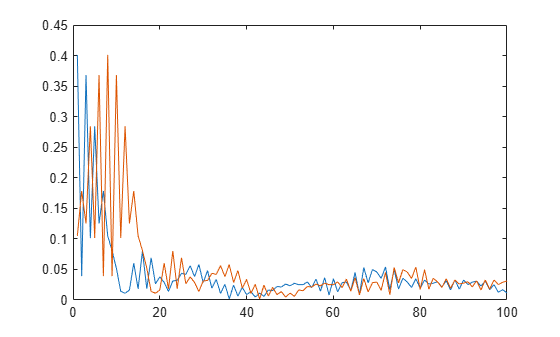# lteULFrameOffsetPUCCH3

PUCCH format 3 DM-RS uplink subframe timing estimate

## Syntax

``offset = lteULFrameOffsetPUCCH3(ue,chs,waveform)``
``````[offset corr] = lteULFrameOffsetPUCCH3(ue,chs,waveform)``````

## Description

example

````offset = lteULFrameOffsetPUCCH3(ue,chs,waveform)` performs synchronization using PUCCH format 3 demodulation reference signals (DM-RS) for the time-domain waveform, `waveform`, given UE-specific settings, `ue`, and PUCCH format 3 configuration, `chs`.The returned value, `offset`, indicates the number of samples from the start of the waveform, `waveform`, to the position in that waveform where the first subframe containing the DM-RS begins.`offset` provides subframe timing; frame timing can be achieved by using `offset` with the subframe number, `ue`.`NSubframe`. This behavior is consistent with real-world operation because the base station knows when, or in which subframe, to expect uplink transmissions.```

example

``````[offset corr] = lteULFrameOffsetPUCCH3(ue,chs,waveform)``` also returns a complex-valued matrix `corr`, which is the signal used to extract the timing offset.```

## Examples

collapse all

Synchronize and demodulate a transmission that has been delayed by seven samples using the PUCCH format 3 demodulation reference signal (DM-RS) symbols.

Initialize configuration structures (`ue` and `pucch3`).

```ue = struct('NULRB',6,'NCellID',0,'NSubframe',0,'Hopping','Off'); ue.CyclicPrefixUL = 'Normal'; ue.NTxAnts = 1; ue.Shortened = 0; pucch3 = struct('ResourceIdx',0);```

On the transmit side, populate `reGrid`, generate `waveform`, and insert a delay of seven samples.

```reGrid = lteULResourceGrid(ue); reGrid(ltePUCCH3DRSIndices(ue,pucch3)) = ltePUCCH3DRS(ue,pucch3); waveform = lteSCFDMAModulate(ue,reGrid); tx = [zeros(7,1); waveform];```

On the receive side, perform synchronization using the PUCCH format 3 DM-RS symbols for the time-domain waveform and demodulate adjusting for the frame timing estimate. Show estimated frame timing offset.

`fOffset = lteULFrameOffsetPUCCH3(ue,pucch3,tx)`
```fOffset = 7 ```
`rxGrid = lteSCFDMADemodulate(ue,tx(1+fOffset:end));`

View the correlation peak for a transmission waveform that has been delayed. The transmission contains PUCCH format 3 demodulation reference signal (DM-RS) symbols available for estimating the waveform timing.

UE Configuration

Create configuration structures for `ue` and pucch3`.`

```ue = struct('NULRB',6,'NCellID',0,'NSubframe',0,'Hopping','Off'); ue.CyclicPrefixUL = 'Normal'; ue.NTxAnts = 1; ue.Shortened = 0; pucch3 = struct('ResourceIdx',0);```

Generate Transmission Waveform

On the transmit side, populate a resource grid and generate a waveform containing PUCCH3 DM-RS.

```reGrid = lteULResourceGrid(ue); reGrid(ltePUCCH3DRSIndices(ue,pucch3)) = ltePUCCH3DRS(ue,pucch3); tx = lteSCFDMAModulate(ue,reGrid);```

Waveform Reception

On the receive side, calculate timing offset using the PUCCH3 DM-RS symbols for the time-domain waveform and return the correlations for the transmit waveform and for a delayed version of the transmit waveform.

```[~,corr] = lteULFrameOffsetPUCCH3(ue,pucch3,tx); txDelayed = [zeros(7,1); tx]; [offset,corrDelayed] = lteULFrameOffsetPUCCH3(ue,pucch3,txDelayed); ```

Plot the correlation data before and after delay is added. Zoom in on the x-axis to view correlation peaks.

```plot(corr) hold on plot(corrDelayed) hold off xlim([0 100])```Correct the timing offset and demodulate the received waveform.

`rrxGrid = lteSCFDMADemodulate(ue,txDelayed(1+offset:end));`

## Input Arguments

collapse all

UE-specific settings, specified as a structure with the following fields.

Parameter FieldRequired or OptionalValuesDescription
`NULRB`Required

Scalar integer from 6 to 110

Number of uplink resource blocks. (${N}_{\text{RB}}^{\text{UL}}$)

`NCellID`Required

Integer from 0 to 503

Physical layer cell identity

`NSubframe`Required

0 (default), nonnegative scalar integer

Subframe number

`CyclicPrefixUL`Optional

`'Normal'` (default), `'Extended'`

Cyclic prefix length

`NTxAnts`Optional

1 (default), 2, 4

Number of transmission antennas.

`Hopping`Optional

`'Off'` (default), `'Group'`

Frequency hopping method.

`Shortened`Optional

0 (default), 1

Option to shorten the subframe by omitting the last symbol, specified as 0 or 1. If `1`, the last symbol of the subframe is not used. For subframes with possible SRS transmission, set `Shortened` to 1 to maintain a standard compliant configuration.

`NPUCCHID`Optional

Integer from 0 to 503

PUCCH virtual cell identity. If this field is not present, `NCellID` is used as the identity.

Data Types: `struct`

PUCCH format 3 configuration, specified as a scalar structure with the following fields.

Parameter FieldRequired or OptionalValuesDescription
`ResourceIdx`Optional

0 (default), integer from 0 to 549, or vector of integers.

PUCCH resource indices which determine the physical resource blocks, cyclic shift, and orthogonal cover used for transmission (${n}_{PUCCH}^{\left(3\right)}$). Define one index for each transmission antenna.

Data Types: `struct`

Time-domain waveform, specified as a numeric matrix. `waveform` must be a NS-by-NR matrix, where NS is the number of time-domain samples and NR is the number of receive antennas. `waveform` should be at least one subframe long and contain the DM-RS signals.

Generate `waveform` by SC-FDMA modulation of a resource matrix using the `lteSCFDMAModulate` function, or by using one of the channel model functions, `lteFadingChannel`, `lteHSTChannel`, or `lteMovingChannel`.

Data Types: `double`
Complex Number Support: Yes

## Output Arguments

collapse all

Number of samples from the start of the waveform to the position in that waveform where the first subframe begins, returned as a scalar integer. `offset` is computed by extracting the timing of the peak of the correlation between `waveform` and internally generated reference waveforms containing DM-RS signals. The correlation is performed separately for each antenna and the antenna with the strongest correlation is used to compute `offset`.

Note

`offset` is the position of `mod(max(abs(corr),LSF))`, where LSF is the subframe length.

Signal used to extract the timing offset, returned as a numeric matrix. `corr` has the same dimensions as `waveform`.

## Version History

Introduced in R2014a